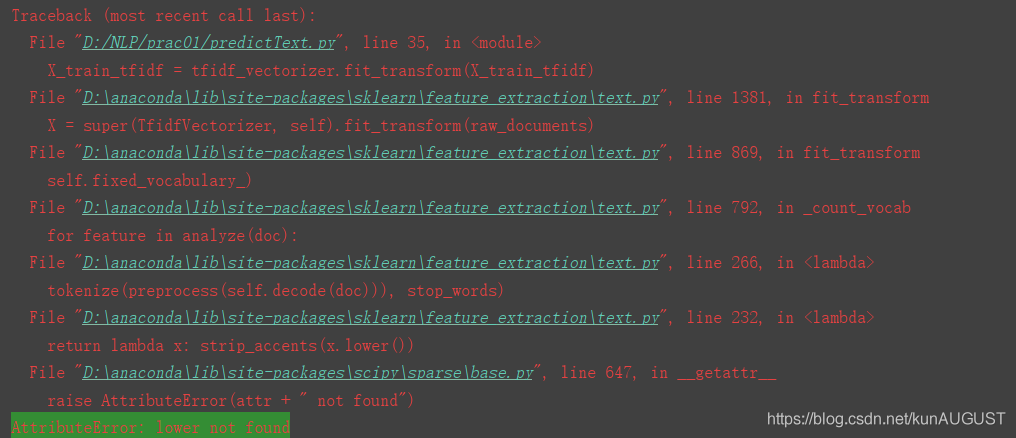# AttributeError: lower not found (NLP extracted tfidf features)The root cause lies in the incorrect use of fit, transform and fit_transform.
First, make it clear that the incoming parameters can be possible causes of series
errors:

When using TfidfVectorizer(), transform after fit_transform

``````def tfidf(X_train):
tfidf_vec = TfidfVectorizer()
X_train_tfidf = tfidf_vec.fit_transform(X_train)
X_train_tfidf = tfidf_vec.transform(X_train_tfidf)
return X_train_tfidf``````

Here the result of fit_transform is passed to transform again, and the transform is repeated

The correct way to use it should be:

``````def tfidf(X_train,X_test):
tfidf_vec = TfidfVectorizer()
X_train_tfidf = tfidf_vec.fit_transform(X_train)
X_test_tfidf = tfidf_vec.transform(X_test)
return X_train_tfidf,X_test_tfidf``````

fit_transform into the training set, transform into the test set

Or you can fit first and then transform

``````def tfidf(data):
tfidf_vec = TfidfVectorizer()
tfidf_model = tfidf_vec.fit(data)
print(tfidf_model.dtype)
X_tfidf = tfidf_model.transform(data)
return X_tfidf``````

You can also use CountVectorizer() and TfidfTransformer()

``````def tfidf(data):
vectorizer = CountVectorizer() # This class will transform the words in the text into a word frequency matrix, and the matrix element a[i][j] represents the word frequency of word j under class i text
transformer = TfidfTransformer() # This class will count the tf-idf weights of each word
tfidf_before = vectorizer.fit_transform(data)
tfidf = transformer.fit_transform(tfidf_before)
return tfidf``````

Note that the first type is divided into training set and test set. It needs to be divided into training set and test set before
inputting parameters. The latter two need to extract tfidf features and then divide training set and test set,
otherwise it may cause training set and test set. The feature dimensions of the set are different, and they are not transformed under the same standard